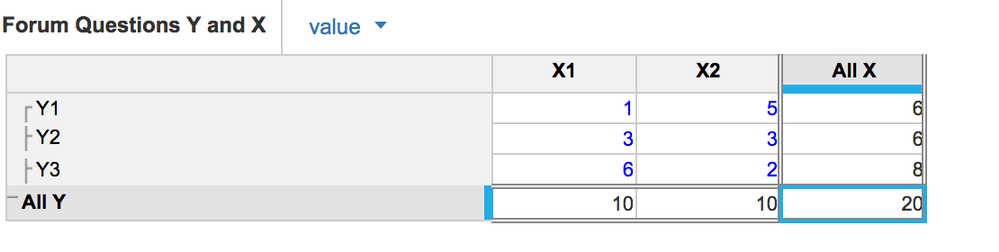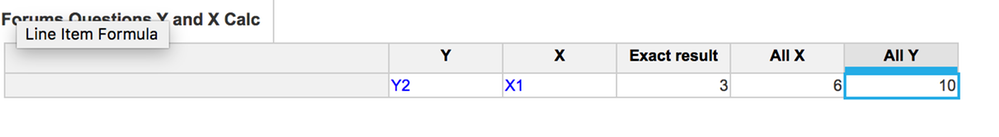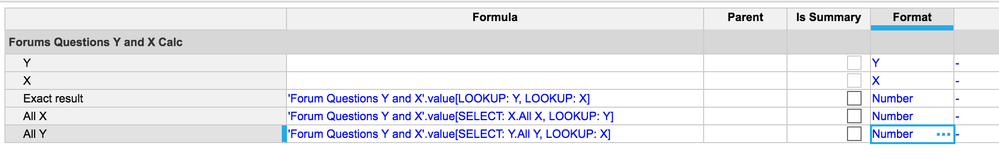# How do I sum across dimensions other than time?Hi All,

I have a question about how to calculate sum cross specific dimension.

For instance, I have a input like this:

X1       X2

Y1   1         5

Y2   3         3

Y3   6         2

Now both X and Y are lists that I created and put into module's page selector so i can toggle.

I would like to create a module to such that when i choose X1 and Y2, there will be a column hold value 3, then another cell hold ALL X's sum within Y2 (6) and another hold ALL Y's sum within X1 (10).

Is there anyway I can achieve this?

Thank you so much!

Tagged:

•Hi,

this is such a great question to understand dimensionality and summary in Anaplan !

I did an example, here's the "database":Note that you need to have summary levels in your dimensions

Then the result:and how this is achieved:LOOKUPs are fully dynamic and they use the user input to find the value value at the crossing of the dimensions

SELECTs are searching for a fixed value ented in the formulas, here the sumary level of the required dimension